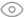# 最前端丨初级开发者福音：手把手教你实现数字滚动效果~96 2023-09-21

• React ：一个用于构建用户界面的代码库。React 组件就像是一个自定义、可重用的 HTML 元素一样，能够帮助开发者快速有效地构建用户界面。

• GSAP：一个业界知名的动画平台，你可以使用 GSAP 为 JavaScript 可以触及的几乎所有内容制作动画，无论您是想要为 UI、SVG、Three.js 还是 React 组件制作动画，GSAP 都能满足您的需求。

Step 1：分析需求

• 该组件接收一个数字用于展示，在这个数字的值改变后，需要展示一个过场动画，并在指定的时间后滚动至新的数字。

• 在动画过程中，如果传入数字再次改变，重新播放滚动效果并滚动至新的数字。

• 如果数字中包含负号、小数点或千分位，符号不进行滚动

Step 2：实现单个数字的滚动效果

# 1、动画分析

• 视图驱动：将动画时间分为两个部分，第一个部分将数字列表滚动 n 圈,第二部分从当前位置缓慢滚动至目标数字，在这整个过程中，数字的滚动速度应该是从一个精准计算的初始速度不断递减直至为零的。

• 数据驱动：预留一个较短的时间播放收尾的动画，用较长的时间以恒定的、较快的速度无限滚动列表，时间到后，不论列表目前滚动到哪个位置，立即将列表移动到目标数字附近并播放一个收尾动画。

# 2、准备静态的 HTML 元素

````const NumberScrollItem = () => {`` const numbers = new Array(10)`` .fill(null)`` .map((_, index) => index)`` .concat(0)`` return (`` `
```
`"number-scroll__stage">`` `
`"number-scroll__gimmick ">`` {numbers.map((number) => (```` ```
`{number}`
``` ```` ))}`` `
` `
` )``}`` `` `

# 3、让数字列表滚动起来

````const COUNT_OF_NUMBERS = 10 // 隐藏在舞台外的数字个数``const HEIGHT_PER_NUMBER = 32 // 每个数字所占高度``const ONE_LAP = HEIGHT_PER_NUMBER * COUNT_OF_NUMBERS // 列表滚动一圈的位移`` ``const useNumberScroll = (dependencies = []) => {`` const ref = useRef()`` `` const animate = () => {`` const gimmicSelector = '.number-scroll__gimmick'`` gsap.fromTo(`` gimmicSelector,`` { y: 0 },`` {`` y: -ONE_LAP,`` duration: 3,`` ease: 'none',`` }`` )`` }`` `` useGSAP({`` animate,`` ref,`` dependencies,`` })`` `` return ref``}`` ``const NumberScrollItem = () => {`` const ref = useNumberScroll()`` return (`` `
```
`"number-scroll__stage" ref={ref}>`` {/* ... */}`` `
` )``}`

``const useGSAP = ({ animate, ref, dependencies = [] }) => {`` return useLayoutEffect(() => {`` let ctx = gsap.context(animate, ref)`` return () => {`` ctx.revert()`` }`` }, dependencies)``}``

gsap.context 指定了动画的上下文，在 animate 函数中书写的选择器都将作用于指定的元素范围内.

# 4、让数字列表可以无限滚动

repeat 属性可以控制动画重复的次数，-1 代表无限次。

``const animate = () => {`` gsap.fromTo(`` gimmicSelector,`` { y: 0 },`` {`` y: -ONE_LAP,`` duration: 3,`` ease: 'none',`` repeat: -1, // 无限重复`` }`` )``}``

# 5、给滚动中的数字加上一层模糊滤镜

``const getScrollSpeed = () => 320 // 设置一个较快的速度即可，单位：px / s``const getDuration = (s, v) => s / v // 时间 = 位移 / 速度`` ``const animate = () => {`` gsap.fromTo(`` gimmicSelector,`` { y: 0 },`` {`` y: -ONE_LAP,`` duration: getDuration(ONE_LAP, getScrollSpeed()),`` ease: 'none',`` repeat: -1, // 无限重复`` onStart() {`` // 添加模糊滤镜`` gsap.set(gimmicSelector, {`` css: {`` filter: 'blur(1px)',`` },`` })`` },`` }`` )``}``

# 6、让数字列表停止滚动

# 视图驱动

• 指定时间后停下：Vο + at = 0；

• 停下时总位移减去必要的位移正好是滚动一圈的整数倍：(s - S1) % S2 = 0；

• 带入位移公式 s = Vοt + at² / 2 可以得到：(Vοt / 2 - S1) % S2 = 0。

# 数据驱动

1）设定一个播放收尾动画的时间，比如总时长的 75% 播放无限滚动，25% 播放收尾动画；

2）通过 onUpdate（每次动画更新时触发）和 this.pause() 控制无限滚动的时间；

3）为 NumberScrollItem 绑定一个 data-value 属性，表示最后滚动到哪个数字；

4）停止无限滚动后：

• 撤除滤镜；

• 通过 HTMLElement.dataset 获取目标数字的值；

• 用剩下的时间播放一个从目标数字上方滚动至目标数字的动画，使用 gsap Eases 中内置的时间函数 elastic.out()（ 类似于 CSS animation-timing-function）使动画效果更有弹性。

````const NumberScrollItem = (props) => {`` const ref = useNumberScroll({`` duration: 5,`` })`` return (`` `
```
`"number-scroll__stage" ref={ref}>`` `
`"number-scroll__gimmick" data-value={0}>`` {/* ... */}`` `
` `
` )``}`` ``const ENDING_ANIMATION_PERCENT = 0.25 // 收尾动画的时间占比`` ``const useNumberScroll = ({ duration }, dependencies = []) => {`` const startTime = useRef() // 用于控制无限滚动的时间`` const endingDuration = min([duration * ENDING_ANIMATION_PERCENT, 2]) // 收尾动画最多播放 2 秒`` const loopDuration = duration - endingDuration`` `` const endAnimate = (ctx) => {`` // 停止无限滚动`` ctx.pause()`` const ele = ctx.targets()`` // 获取目标数字的值`` const value = +ele.dataset.value`` const valueOffset = value === 0 ? 10 : value`` // 撤除滤镜`` gsap.set(ele, {`` css: {`` filter: '',`` },`` })`` // 播放一个从目标数字上方滚动至目标数字的动画`` gsap.fromTo(`` ele,`` {`` y: -((valueOffset - 1) * HEIGHT_PER_NUMBER), // 目标数字的上一个数字`` },`` {`` y: -(valueOffset * HEIGHT_PER_NUMBER),`` duration: endingDuration,`` ease: 'elastic.out(1, 0.3)',`` }`` )`` }`` `` const animate = () => {`` gsap.fromTo(`` gimmicSelector,`` { y: 0 },`` {`` onStart() {`` startTime.current = Date.now()`` },`` onUpdate() {`` const isEnd = Date.now() - startTime.current >= loopDuration * 1000`` if (isEnd) endAnimate(this)`` },`` }`` )`` }``}`

Step 3：组件接口设计

``NumberScroll.propTypes = {`` number: PropTypes.oneOfType([PropTypes.number, PropTypes.string]),`` duration: PropTypes.number,``}`` ``NumberScroll.defaultProps = {`` number: 0,`` duration: 5,``}``

````function App() {`` return (`` `
```
`"App">`` '1,231,232.00'} duration={5} />`` `
` )``}`

Step 4：完善组件

# 支持多位数

1）将传入数字拆分为一个数组；

2）将数组渲染为多个；

3）将参数 value 绑定至 data-value。

````const NumberScroll = (props) => {`` const numbers = String(props.number).split('')`` `` return (`` `
```
` {numbers.map((item) => (`` `` ))}`` `
` )``}`` ``const NumberScrollItem = (props) => {`` return (`` `
` `
` {/* ... */}`` `
` `
` )``}`` `

# 支持负数、浮点数、千分位数字

1）新建组件 SymbolItem 用于显示符号；

2）添加判断数字的方法；

3）条件渲染不同的组件。

````const SymbolItem = (props) => {`` const { value } = props`` `` return (`` `
```
`"number-scroll__stage">```` ```
`"number-scroll__gimmick ">{value}`
``` ```` `
` )``}`` ``const isNumber = (value) => /^\d\$/g.test(value)`` ``const NumberScroll = (props) => {`` return (`` `
`"number-scroll">`` {numbers.map((item) =>`` isNumber(item) ? (`` `` ) : (`` `` )`` )}`` `
` )``}`

# 支持在传入

1）将 useNumberScroll 从提升至

（避免 props.number 更改时，某位数的值和更改前一样，导致个别数字没有触发动画）；

2）将 props.number 作为触发动画的依赖项；

3）为添加一个 className 以区分数字和符号；

4）传给 gsap.fromTo 的选择器只选择类型为数字的子项，使用 ctx.targets().forEach() 逐个为选中的元素添加结尾动画。

````const NumberScroll = (props) => {`` // 提升至父组件`` const ref = useNumberScroll(`` {`` duration: props.duration,`` },`` [props.number]`` )`` `` return (`` `
```
`"number-scroll" ref={ref}>`` {/* ... */}`` `
` )``}`` ``const NumberScrollItem = (props) => {`` return (`` `
`"number-scroll__stage">`` `
` className="number-scroll__gimmick number-scroll__gimmick--scroll"`` data-value={props.value}`` style={{`` transform: `translateY(-\${props.value * HEIGHT_PER_NUMBER}px)`,`` }}`` >`` {/* ... */}`` `
` `` )``}`` ``const endAnimate = (ctx) => {`` // 为所有类型为数字的子项添加收尾动画`` ctx.targets().forEach((ele) => {`` const value = +ele.dataset.value`` // end animate`` })``}`` ``const animate = () => {`` // 只选择类型为数字的子项`` const gimmicSelector = '.number-scroll__gimmick--scroll'`` gsap`` .fromTo`` // ...`` ()``}`

133 篇文章